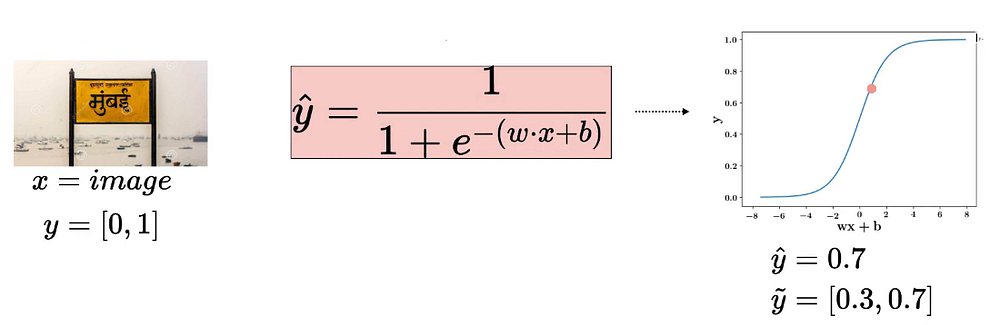# Sigmoid Neuron and Cross-Entropy

Source: Deep Learning on Medium# Sigmoid Neuron and Cross-Entropy

This article covers the content discussed in the Sigmoid Neuron and Cross-Entropy module of the Deep Learning course and all the images are taken from the same module.

The situation that we have is that we are given an image, we know the true label for that image if it contains text or not and in the below case since the image contains text, we can say that all the probability mass is on the random variable taking on the value 1 and there is 0 probability mass on the random variable taking on the value No Text.

Of course, in practice, we don’t know this true distribution, so we are approximating the same using the sigmoid function, and when we pass this input as x to the sigmoid neuron, we get the output to say 0.7 which we can again interpret as the probability distribution as the probability of the image containing text is 0.7 and the probability of the image not containing text is 0.3.

So, we were computing the difference between these two distributions using the squared error loss but now we have a better metric, something which is grounded in probability theory which is the KL Divergence between these two distributions.